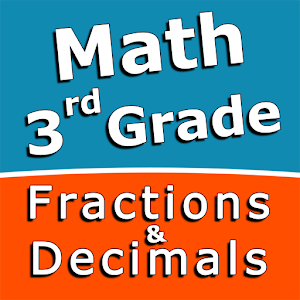# Third grade Math skills – Fractions and DecimalsRating: 0/5

Intuitive interface powered by handwriting input and a choice of three fun and engaging mini games in addition to a regular math trainer mode make our app stand out from the crowd of generic math learning apps.

With Third grade Math skills – Fractions and Decimals you can practice and improve the following math skills:
– Add fractions with like denominators
– Subtract fractions with like denominators
– Write fractions in lowest terms
– Add decimal numbers
– Subtract decimal numbers
– Add three decimal numbers
– Convert decimals to fractions and mixed numbers
– Convert fractions and mixed numbers to decimals

Choose between four different modes:
– Math Trainer
– Math Shot mini-game
– Math Gravity mini-game
– Math Trick Shot mini-game
Download it here### Words Everywhere PRO